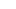# ‘Equating and Scaling Score’ Made Easy

The different test forms on different dates may lead to inequality in terms of difficulty. Equating is a statistical process, used to adjust scores on the basis of difficulty level.

In an objective type test, raw scores include scores earned by an examinee by marking correct or incorrect options. These raw scores are transformed to scale scores. The raw to scale score transformation can be chosen by test developers.

This is done by using linear equation method.

Linear equating: This method used for the test forms vary in difficulty terms and allows score to scale. To consider this method mathematically, we take two different test forms X and Y. The linear conversion is mathematically defined by

Where

X = Raw score of a student in test form X

Y = Raw score of a student in test form Y

mean(X) and mean(Y) are the mean of all raw scores of test form X and Y respectively

SD(X) and SD(Y) are the standard deviation of all raw scores of test form X and Y respectively.

Solving above equation for the score in test form Y will give us a formula for adjusting any given raw score X on the test form YThe adjusted scores of the form X will have the same mean and standard deviation as the raw scores on the form Y.

To obtain scale scores again linear conversion is used.Where

sc(Y) = scale score of raw score Y in test form Y.

mean(sc) and SD(sc) are mean and standard deviation of scale scores. The testing agency chooses constant value for these. For example, IBPS used mean(sc) = 25 and SD(sc) = 5 for CWE-POs/MTs-II.

Let us consider above method through an example:

Assume two test forms X and Y of maximum score 50.On calculating mean and standard deviation for form X and Y, we have

Mean(X) = 22, SD(X) = 3.29

Mean(Y) = 24, SD(Y) = 2

Using equation 1, we have adjusted raw score X on the test Y as below:Now using equation 2, convert above equated scores to scale scores.

Use mean(sc) = 25, SD(sc) = 5Please note that ‘0 to 50 scale’ is used in this example. Generally testing agencies use different scale from maximum marks to avoid confusion. IBPS uses same scale as maximum marks but for CAT, IIMs use different scale (0 to 225 for each section).